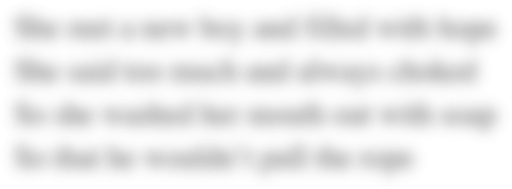# M&M lab Physics

When you performed Step 2 of the procedure, you actually made a cylinder of M&M'S®. The cylinder was rather "smushed," and the height of the cylinder was the thickness of an M&M'S®. Recall that the equation for the volume of a cylinder is V = (3.14)r2h.

Rearrange the equation for "h." Show your work.

Using the data from Table 1 and your equation, calculate the average thickness (height) of an M&M'S® for each trial. Record your calculated values in Table 1. Hint: Students often forget that they must use the radius, and not the diameter, in the equation. Copy Table 1 into the assignment.

You now have two values for the thickness of an M&M'S® in Table 1. Determine the average M&M'S® thickness using these values and record your value in Table 3.

You have just determined a value for the thickness of an M&M'S® using the indirect method. What makes this method "indirect"?
Field of study:
Date Due:
Thursday, September 6, 2018